# How to Minimize Customer ChurnPhoto by Louis Hansel

## Context

Predict behavior to retain customers. You can analyze all relevant customer data and develop focused customer retention programs.

## Content

Each row represents a customer, each column contains customer’s attributes described on the column Metadata.

The data set includes information about:

• Customers who left within the last month – the column is called Churn
• Services that each customer has signed up for – phone, multiple lines, internet, online security, online backup, device protection, tech support, and streaming TV and movies
• Customer account information – how long they’ve been a customer, contract, payment method, paperless billing, monthly charges, and total charges
• Demographic info about customers – gender, age range, and if they have partners and dependents

## First insight

``````import numpy as np
import pandas as pd
import matplotlib.pyplot as plt
import seaborn as sns
``````
``````from sklearn.decomposition import PCA
from sklearn.preprocessing import StandardScaler, MinMaxScaler
from sklearn.model_selection import train_test_split
``````
``````from sklearn.metrics import f1_score, classification_report

from sklearn.model_selection import cross_val_score
from sklearn.linear_model import LogisticRegression, SGDClassifier
from sklearn.svm import SVC, LinearSVC
``````
``````import lightgbm as lgbm
import xgboost as xgb
``````
``````import warnings
warnings.simplefilter(action='ignore', category=FutureWarning)

pd.set_option('display.max_columns', 100)
``````
``````df = pd.read_csv('./input/Telco-Customer-Churn.csv')
``````
customerID gender SeniorCitizen Partner Dependents tenure PhoneService MultipleLines InternetService OnlineSecurity OnlineBackup DeviceProtection TechSupport StreamingTV StreamingMovies Contract PaperlessBilling PaymentMethod MonthlyCharges TotalCharges Churn
0 7590-VHVEG Female 0 Yes No 1 No No phone service DSL No Yes No No No No Month-to-month Yes Electronic check 29.85 29.85 No
1 5575-GNVDE Male 0 No No 34 Yes No DSL Yes No Yes No No No One year No Mailed check 56.95 1889.5 No
2 3668-QPYBK Male 0 No No 2 Yes No DSL Yes Yes No No No No Month-to-month Yes Mailed check 53.85 108.15 Yes
3 7795-CFOCW Male 0 No No 45 No No phone service DSL Yes No Yes Yes No No One year No Bank transfer (automatic) 42.30 1840.75 No
4 9237-HQITU Female 0 No No 2 Yes No Fiber optic No No No No No No Month-to-month Yes Electronic check 70.70 151.65 Yes
``````df.shape
``````
``````(7043, 21)
``````

The dataset contains about 7000 customers with 19 features.

Features are the following:

• `customerID`: a unique ID for each customer
• `gender`: the gender of the customer
• `SeniorCitizen`: whether the customer is a senior (i.e. older than 65) or not
• `Partner`: whether the customer has a partner or not
• `Dependents`: whether the customer has people to take care of or not
• `tenure`: the number of months the customer has stayed
• `PhoneService`: whether the customer has a phone service or not
• `MultipleLines`: whether the customer has multiple telephonic lines or not
• `InternetService`: the kind of internet services the customer has (DSL, Fiber optic, no)
• `OnlineSecurity`: what online security the customer has (Yes, No, No internet service)
• `OnlineBackup`: whether the customer has online backup file system (Yes, No, No internet service)
• `DeviceProtection`: Whether the customer has device protection or not (Yes, No, No internet service)
• `TechSupport`: whether the customer has tech support or not (Yes, No, No internet service)
• `StreamingTV`: whether the customer has a streaming TV device (e.g. a TV box) or not (Yes, No, No internet service)
• `StreamingMovies`: whether the customer uses streaming movies (e.g. VOD) or not (Yes, No, No internet service)
• `Contract`: the contract term of the customer (Month-to-month, One year, Two year)
• `PaperlessBilling`: Whether the customer has electronic billing or not (Yes, No)
• `PaymentMethod`: payment method of the customer (Electronic check, Mailed check, Bank transfer (automatic), Credit card (automatic))
• `MonthlyCharges`: the amount charged to the customer monthly
• `TotalCharges`: the total amount the customer paid

And the Target :

• `Churn`: whether the customer left or not (Yes, No)

As you can see, many features are categorical with more than 2 values. You will have to handle this.

Take time to make a proper and complete EDA: this will help you build a better model.

# Exploratory Data Analysis¶

Global infos on the dataset (null values, types…)

``````df.info()
``````
``````<class 'pandas.core.frame.DataFrame'>
RangeIndex: 7043 entries, 0 to 7042
Data columns (total 21 columns):
customerID          7043 non-null object
gender              7043 non-null object
SeniorCitizen       7043 non-null int64
Partner             7043 non-null object
Dependents          7043 non-null object
tenure              7043 non-null int64
PhoneService        7043 non-null object
MultipleLines       7043 non-null object
InternetService     7043 non-null object
OnlineSecurity      7043 non-null object
OnlineBackup        7043 non-null object
DeviceProtection    7043 non-null object
TechSupport         7043 non-null object
StreamingTV         7043 non-null object
StreamingMovies     7043 non-null object
Contract            7043 non-null object
PaperlessBilling    7043 non-null object
PaymentMethod       7043 non-null object
MonthlyCharges      7043 non-null float64
TotalCharges        7043 non-null object
Churn               7043 non-null object
dtypes: float64(1), int64(2), object(18)
memory usage: 1.1+ MB
``````

Nb of each type

``````df.dtypes.value_counts()
``````
``````object     18
int64       2
float64     1
dtype: int64
``````

Nb of unique value for each type

``````df.select_dtypes('object').apply(pd.Series.nunique, axis = 0)
``````
``````customerID          7043
gender                 2
Partner                2
Dependents             2
PhoneService           2
MultipleLines          3
InternetService        3
OnlineSecurity         3
OnlineBackup           3
DeviceProtection       3
TechSupport            3
StreamingTV            3
StreamingMovies        3
Contract               3
PaperlessBilling       2
PaymentMethod          4
TotalCharges        6531
Churn                  2
dtype: int64
``````

## Target infos

``````df['Churn'].value_counts()
``````
``````No     5174
Yes    1869
Name: Churn, dtype: int64
``````
``````df['Churn'].str.replace('No', '0').str.replace('Yes', '1').astype(int).plot.hist()
``````
``````<matplotlib.axes._subplots.AxesSubplot at 0x7f4a41ebe438>
``````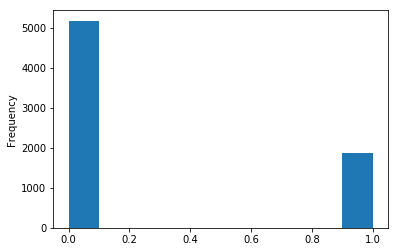Basic stats on numerical cols

``````df.describe()
``````
SeniorCitizen tenure MonthlyCharges
count 7043.000000 7043.000000 7043.000000
mean 0.162147 32.371149 64.761692
std 0.368612 24.559481 30.090047
min 0.000000 0.000000 18.250000
25% 0.000000 9.000000 35.500000
50% 0.000000 29.000000 70.350000
75% 0.000000 55.000000 89.850000
max 1.000000 72.000000 118.750000

## Basic cleaning

``````df.duplicated().sum()
``````
``````0
``````
``````df.isnull().sum()
``````
``````customerID          0
gender              0
SeniorCitizen       0
Partner             0
Dependents          0
tenure              0
PhoneService        0
MultipleLines       0
InternetService     0
OnlineSecurity      0
OnlineBackup        0
DeviceProtection    0
TechSupport         0
StreamingTV         0
StreamingMovies     0
Contract            0
PaperlessBilling    0
PaymentMethod       0
MonthlyCharges      0
TotalCharges        0
Churn               0
dtype: int64
``````
``````df = df.drop(columns=['customerID'])
``````

No missing or duplicated rows. The customer ID is irrelevant and can be dropped.

## Dealing with abnormal values

The ‘TotalCharges’ column has an object type, but it is supposed to contain only numerical values…Let’s dig a little deeper:

``````# example for the record strip non digit values
#test = pd.Series(["U\$ 192.01"])
#test.str.replace('^[^\d]*', '').astype(float)

#df.TotalCharges = df.TotalCharges.str.replace('^[^\d]*', '')
``````
``````df.iloc[0, df.columns.get_loc("TotalCharges")]
``````
``````'29.85'
``````
``````float(df.iloc[0, df.columns.get_loc("TotalCharges")])
``````
``````29.85
``````
``````df.iloc[488, df.columns.get_loc("TotalCharges")]
``````
``````' '
``````
``````len(df[df['TotalCharges'] == ' '])
``````
``````11
``````

Drop strange/missing values (the pandas method to_numeric could also has been used!):

``````# replace missing values by 0
df.TotalCharges = df.TotalCharges.replace(" ",np.nan)

# drop missing values - side note: it represents only 11 out of 7043 rows which is not significant...
df = df.dropna()

# now we can convert the column type
df.TotalCharges = df.TotalCharges.astype('float')

df.shape
``````
``````(7032, 20)
``````
``````num_feat = df.select_dtypes(include=['float', 'int']).columns.tolist()
num_feat.remove('SeniorCitizen')    # SeniorCitizen is only a boolean
num_feat
``````
``````['tenure', 'MonthlyCharges', 'TotalCharges']
``````
``````sns.pairplot(data=df[num_feat])
plt.show()
``````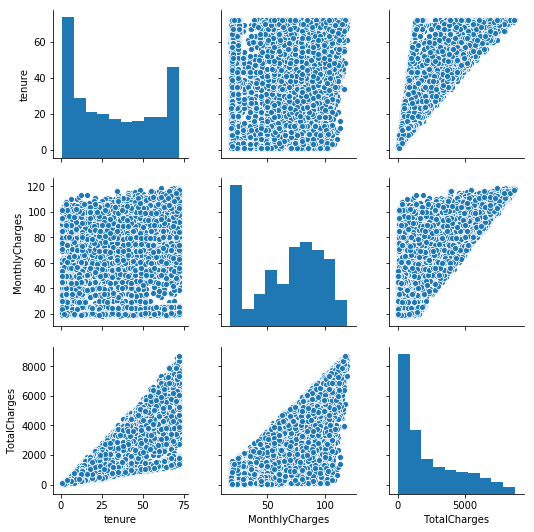Plot distribution of those feat, w/ & w/o the distinction between the customers who churn

``````plt.figure(figsize=(16, 10))

plt.subplot(2, 3, 1)
sns.distplot(df['tenure'])
plt.title('tenure')

plt.subplot(2, 3, 2)
sns.distplot(df['MonthlyCharges'])
plt.title('MonthlyCharges')

plt.subplot(2, 3, 3)
sns.distplot(df['TotalCharges'])
plt.title('TotalCharges')

plt.subplot(2, 3, 4)
sns.kdeplot(df.loc[df['Churn'] == 'No', 'tenure'], shade=True,label = 'Churn == 0')
sns.kdeplot(df.loc[df['Churn'] == 'Yes', 'tenure'], shade=True,label = 'Churn == 1')

plt.subplot(2, 3, 5)
sns.kdeplot(df.loc[df['Churn'] == 'No', 'MonthlyCharges'], shade=True,label = 'Churn == 0')
sns.kdeplot(df.loc[df['Churn'] == 'Yes', 'MonthlyCharges'], shade=True,label = 'Churn == 1')

plt.subplot(2, 3, 6)
sns.kdeplot(df.loc[df['Churn'] == 'No', 'TotalCharges'], shade=True,label = 'Churn == 0')
sns.kdeplot(df.loc[df['Churn'] == 'Yes', 'TotalCharges'], shade=True,label = 'Churn == 1')

``````
``````<matplotlib.axes._subplots.AxesSubplot at 0x7f4a40662e10>
``````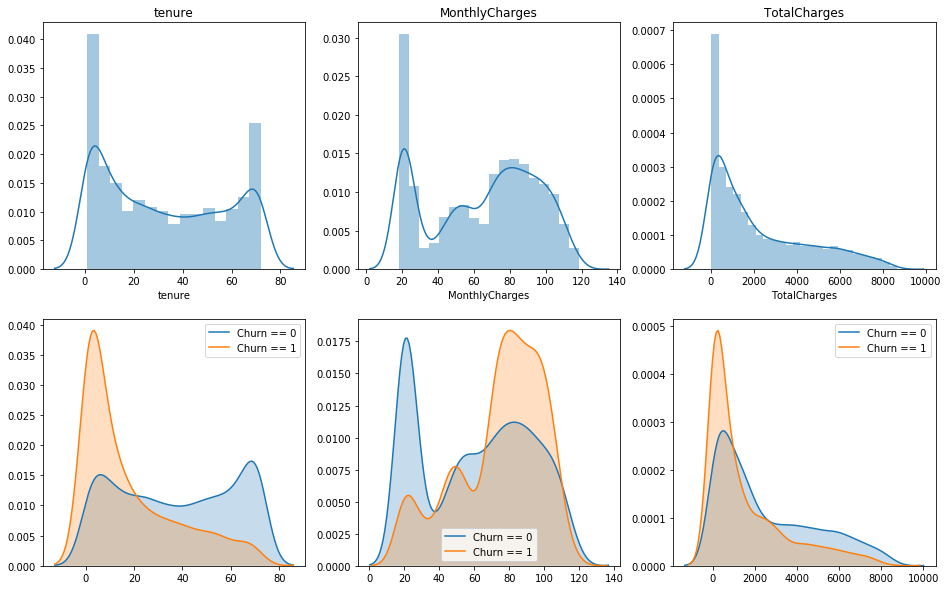Are there any correlations ?

``````corr = df.corr()
corr
``````
SeniorCitizen tenure MonthlyCharges TotalCharges
SeniorCitizen 1.000000 0.015683 0.219874 0.102411
tenure 0.015683 1.000000 0.246862 0.825880
MonthlyCharges 0.219874 0.246862 1.000000 0.651065
TotalCharges 0.102411 0.825880 0.651065 1.000000
``````# Generate a mask for the upper triangle

# Set up the matplotlib figure
f, ax = plt.subplots(figsize=(6, 4))

# Generate a custom diverging colormap
cmap = sns.diverging_palette(220, 10, as_cmap=True)

# Draw the heatmap with the mask and correct aspect ratio
square=True, linewidths=.5, cbar_kws={"shrink": .5}, annot=True)
``````
``````<matplotlib.axes._subplots.AxesSubplot at 0x7f4a401d0da0>
``````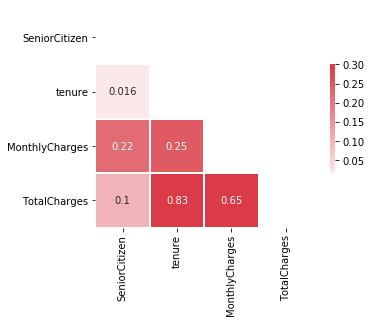``````plt.figure(figsize=(12, 6))

corr = df.apply(lambda x: pd.factorize(x)).corr()
ax = sns.heatmap(corr, xticklabels=corr.columns, yticklabels=corr.columns,
linewidths=.2, cmap="YlGnBu")
``````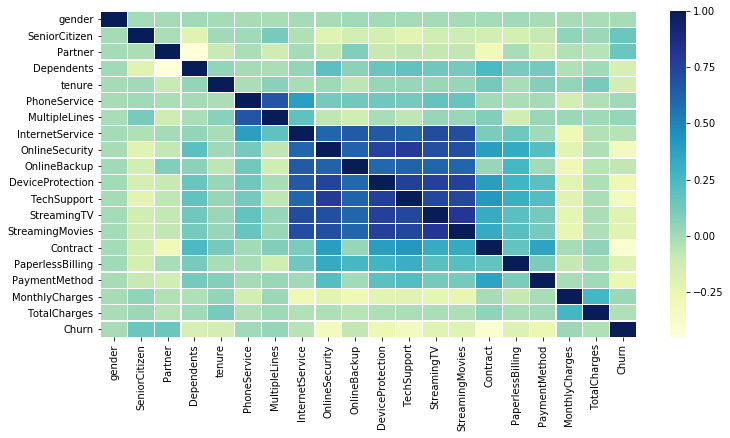``````for c in num_feat:
plt.figure(figsize=(12, 1))
sns.boxplot(df[c])
plt.title(c)
plt.show()
``````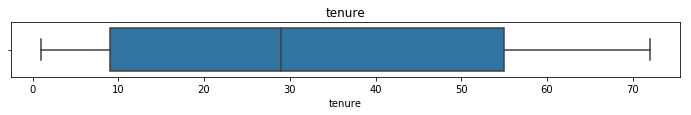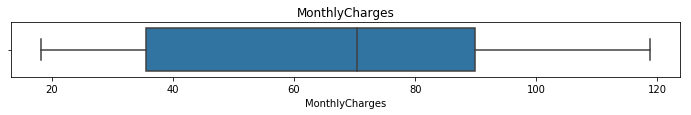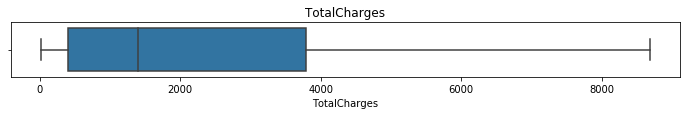``````cat_features = df.select_dtypes('object').columns.tolist()
cat_features
``````
``````['gender',
'Partner',
'Dependents',
'PhoneService',
'MultipleLines',
'InternetService',
'OnlineSecurity',
'OnlineBackup',
'DeviceProtection',
'TechSupport',
'StreamingTV',
'StreamingMovies',
'Contract',
'PaperlessBilling',
'PaymentMethod',
'Churn']
``````

Plot the count of different categories for the other features (with text)

``````plt.figure(figsize=(16, 20))

for i in range(len(cat_features)):
plt.subplot(6, 3, i+1)
sns.countplot(df[cat_features[i]])
#plt.title(cat_features[i])

plt.show()
``````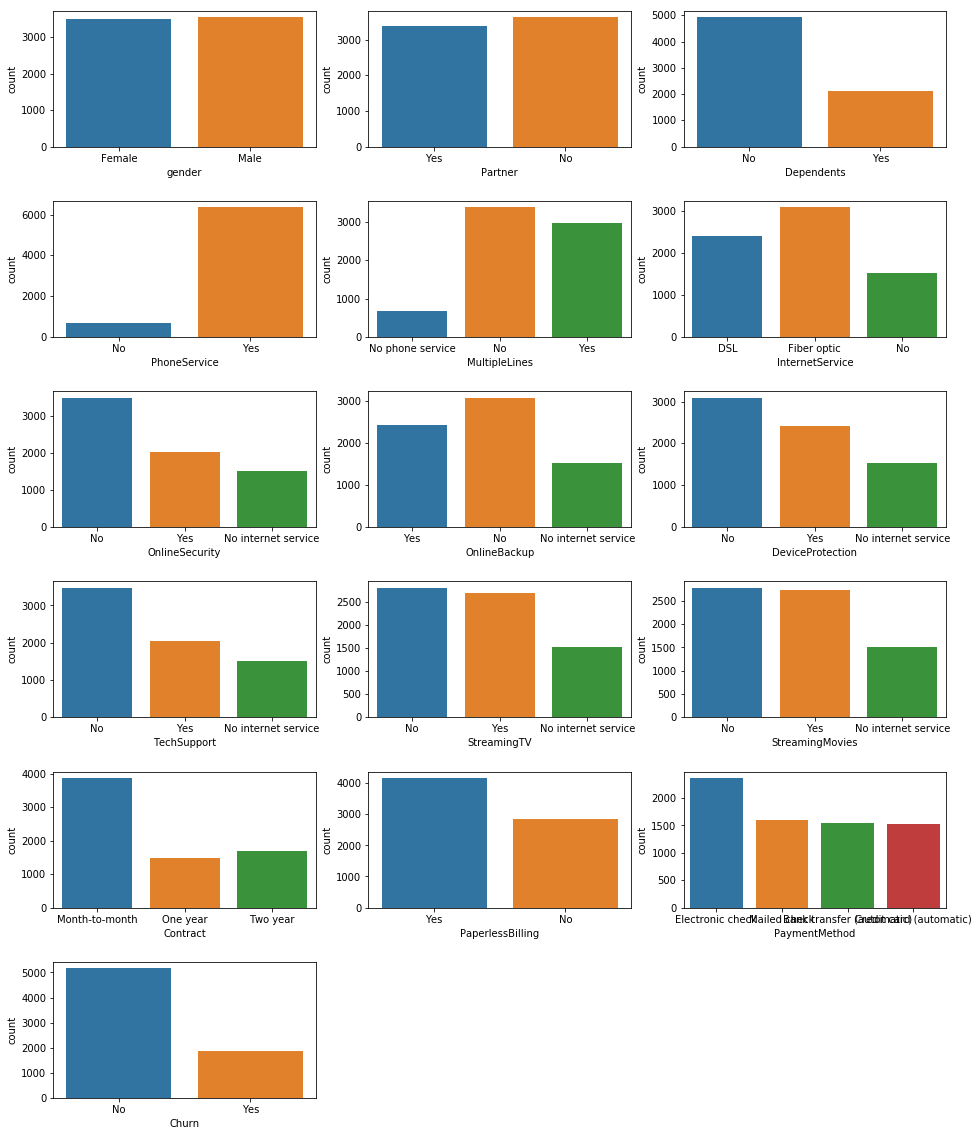Same plot but with the distinction between customers who churn

``````cat_features.remove('Churn')

plt.figure(figsize=(16, 20))

for i in range(len(cat_features)):
plt.subplot(6, 3, i+1)
sns.countplot(df[cat_features[i]], hue=df['Churn'])
#plt.title(cat_features[i])

plt.show()
``````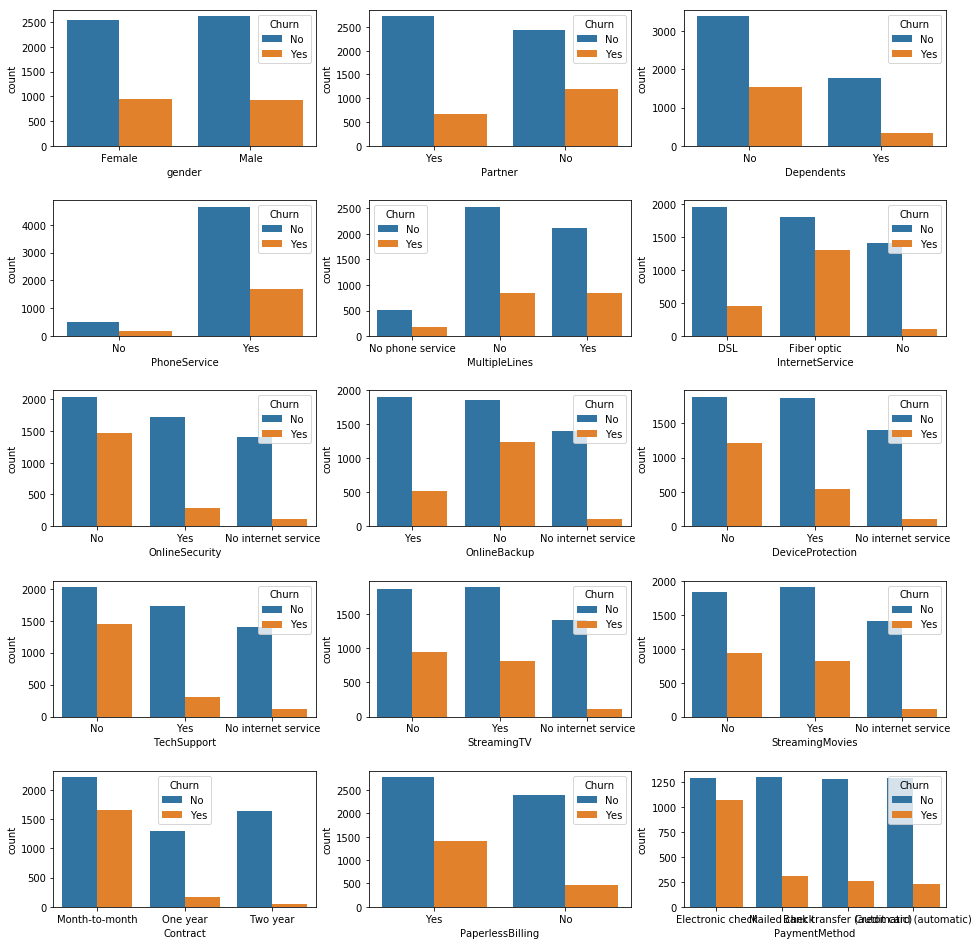# Data Preparation & Feature engineering

Target creation

``````y = df.Churn.str.replace('No', '0').str.replace('Yes', '1').astype(int)
``````

Label encoding of categorical features

``````X = pd.get_dummies(data=df, columns=cat_features, drop_first=True)
X = X.drop(columns=['Churn'])
``````
``````X.shape, y.shape
``````
``````((7032, 30), (7032,))
``````

Features creation

• In this case, it’s complicated to add features from an other dataset because no information is provided with the CSV file we’re using.
• All columns except the user_id are relevant, so all of them are kept.
• We can combine features to create new ones : by dividing TotalCharges with the tenure which provide a kind of charge average per month. This value compared to the Monthly charges can give an idea of the charges’ evolution with time.
``````X['average_charges'] = X['TotalCharges'] / X['tenure']
X.loc[X['tenure'] == 0, 'average_charges'] = X['MonthlyCharges']
``````
SeniorCitizen tenure MonthlyCharges TotalCharges gender_Male Partner_Yes Dependents_Yes PhoneService_Yes MultipleLines_No phone service MultipleLines_Yes InternetService_Fiber optic InternetService_No OnlineSecurity_No internet service OnlineSecurity_Yes OnlineBackup_No internet service OnlineBackup_Yes DeviceProtection_No internet service DeviceProtection_Yes TechSupport_No internet service TechSupport_Yes StreamingTV_No internet service StreamingTV_Yes StreamingMovies_No internet service StreamingMovies_Yes Contract_One year Contract_Two year PaperlessBilling_Yes PaymentMethod_Credit card (automatic) PaymentMethod_Electronic check PaymentMethod_Mailed check average_charges
0 0 1 29.85 29.85 0 1 0 0 1 0 0 0 0 0 0 1 0 0 0 0 0 0 0 0 0 0 1 0 1 0 29.850000
1 0 34 56.95 1889.50 1 0 0 1 0 0 0 0 0 1 0 0 0 1 0 0 0 0 0 0 1 0 0 0 0 1 55.573529
2 0 2 53.85 108.15 1 0 0 1 0 0 0 0 0 1 0 1 0 0 0 0 0 0 0 0 0 0 1 0 0 1 54.075000
3 0 45 42.30 1840.75 1 0 0 0 1 0 0 0 0 1 0 0 0 1 0 1 0 0 0 0 1 0 0 0 0 0 40.905556
4 0 2 70.70 151.65 0 0 0 1 0 0 1 0 0 0 0 0 0 0 0 0 0 0 0 0 0 0 1 0 1 0 75.825000

Scaling data

``````num_feat.append('average_charges')
scaler = MinMaxScaler()
X[num_feat] = scaler.fit_transform(X[num_feat])
``````
``````/home/sunflowa/anaconda3/lib/python3.7/site-packages/sklearn/preprocessing/data.py:323: DataConversionWarning: Data with input dtype int64, float64 were all converted to float64 by MinMaxScaler.
return self.partial_fit(X, y)
``````
``````X.head()
``````
SeniorCitizen tenure MonthlyCharges TotalCharges gender_Male Partner_Yes Dependents_Yes PhoneService_Yes MultipleLines_No phone service MultipleLines_Yes InternetService_Fiber optic InternetService_No OnlineSecurity_No internet service OnlineSecurity_Yes OnlineBackup_No internet service OnlineBackup_Yes DeviceProtection_No internet service DeviceProtection_Yes TechSupport_No internet service TechSupport_Yes StreamingTV_No internet service StreamingTV_Yes StreamingMovies_No internet service StreamingMovies_Yes Contract_One year Contract_Two year PaperlessBilling_Yes PaymentMethod_Credit card (automatic) PaymentMethod_Electronic check PaymentMethod_Mailed check average_charges
0 0 0.000000 0.115423 0.001275 0 1 0 0 1 0 0 0 0 0 0 1 0 0 0 0 0 0 0 0 0 0 1 0 1 0 0.149361
1 0 0.464789 0.385075 0.215867 1 0 0 1 0 0 0 0 0 1 0 0 0 1 0 0 0 0 0 0 1 0 0 0 0 1 0.388372
2 0 0.014085 0.354229 0.010310 1 0 0 1 0 0 0 0 0 1 0 1 0 0 0 0 0 0 0 0 0 0 1 0 0 1 0.374448
3 0 0.619718 0.239303 0.210241 1 0 0 0 1 0 0 0 0 1 0 0 0 1 0 1 0 0 0 0 1 0 0 0 0 0 0.252084
4 0 0.014085 0.521891 0.015330 0 0 0 1 0 0 1 0 0 0 0 0 0 0 0 0 0 0 0 0 0 0 1 0 1 0 0.576539

Splitting train and test sets

``````X_train, X_test, y_train, y_test = train_test_split(X, y, test_size=0.2)
``````

Features importances

``````rnd_clf = RandomForestClassifier(n_estimators=500, n_jobs=-1)
rnd_clf.fit(X, y)
``````
``````RandomForestClassifier(bootstrap=True, class_weight=None, criterion='gini',
max_depth=None, max_features='auto', max_leaf_nodes=None,
min_impurity_decrease=0.0, min_impurity_split=None,
min_samples_leaf=1, min_samples_split=2,
min_weight_fraction_leaf=0.0, n_estimators=500, n_jobs=-1,
oob_score=False, random_state=None, verbose=0,
warm_start=False)
``````
``````feature_importances = pd.DataFrame(rnd_clf.feature_importances_, index = X.columns,
columns=['importance']).sort_values('importance', ascending=False)
feature_importances[:10]
``````
importance
TotalCharges 0.167416
tenure 0.150908
average_charges 0.137030
MonthlyCharges 0.134679
PaymentMethod_Electronic check 0.034640
InternetService_Fiber optic 0.034376
Contract_Two year 0.032602
gender_Male 0.024216
OnlineSecurity_Yes 0.022954
PaperlessBilling_Yes 0.022600
``````plt.figure(figsize=(8, 10))
sns.barplot(x="importance", y=feature_importances.index, data=feature_importances)
plt.show()
``````# Baselines

``````# f1_score binary by default
def get_f1_scores(clf, model_name):
y_train_pred, y_pred = clf.predict(X_train), clf.predict(X_test)
print(model_name, f'\t - Training F1 score = {f1_score(y_train, y_train_pred) * 100:.2f}% / Test F1 score = {f1_score(y_test, y_pred)  * 100:.2f}%')
``````
``````model_list = [RandomForestClassifier(),
LogisticRegression(),
SVC(),
LinearSVC(),
SGDClassifier(),
lgbm.LGBMClassifier(),
xgb.XGBClassifier()
]
``````
``````model_names = [str(m)[:str(m).index('(')] for m in model_list]
``````
``````for model, name in zip(model_list, model_names):
model.fit(X_train, y_train)
get_f1_scores(model, name)
``````
``````RandomForestClassifier 	 - Training F1 score = 95.88% / Test F1 score = 51.62%
LogisticRegression 	 - Training F1 score = 60.45% / Test F1 score = 56.12%
SVC 	 - Training F1 score = 58.92% / Test F1 score = 54.46%
LinearSVC 	 - Training F1 score = 59.91% / Test F1 score = 56.36%
SGDClassifier 	 - Training F1 score = 40.85% / Test F1 score = 36.83%
LGBMClassifier 	 - Training F1 score = 76.50% / Test F1 score = 57.02%
XGBClassifier 	 - Training F1 score = 62.61% / Test F1 score = 58.36%
``````

The 1st model - RandomForrest Clf - is clearly overfitting the train dataset and can’t generalize. The others models don’t have good results and are probably underfitting. So let’s tuned them !

# Training more accurately other models

## Randomforest with weighted classes

``````rfc = RandomForestClassifier()
rfc.fit(X_train, y_train)
get_f1_scores(rfc, 'RandomForest')
``````
``````RandomForest 	 - Training F1 score = 96.83% / Test F1 score = 48.52%
``````
``````y.sum(), len(y) - y.sum()
``````
``````(1869, 5163)
``````
``````rfc = RandomForestClassifier(class_weight={1:1869, 0:5174})
rfc.fit(X_train, y_train)
get_f1_scores(rfc, 'RandomForest weighted')
``````
``````RandomForest weighted 	 - Training F1 score = 96.35% / Test F1 score = 51.89%
``````

The improvement is not significant…

## LGBM with weighted classes

``````lgbm_w = lgbm.LGBMClassifier(n_jobs = -1, class_weight={0:1869, 1:5174})
lgbm_w.fit(X_train, y_train)
get_f1_scores(lgbm_w, 'LGBM weighted')
``````
``````LGBM weighted 	 - Training F1 score = 78.76% / Test F1 score = 63.03%
``````

## XGB with ratio

``````ratio = ((len(y) - y.sum()) - y.sum()) / y.sum()
ratio
``````
``````1.7624398073836276
``````
``````xgb_model = xgb.XGBClassifier(objective="binary:logistic", scale_pos_weight=ratio)
xgb_model.fit(X_train, y_train)
get_f1_scores(xgb_model, 'XGB with ratio')
``````
``````XGB with ratio 	 - Training F1 score = 66.48% / Test F1 score = 63.47%
``````

That’s a little better.

``````abc = AdaBoostClassifier()
abc.fit(X_train, y_train)
``````
``````Adaboost 	 - Training F1 score = 60.75% / Test F1 score = 58.05%
``````

# Using GridsearchCV & Combining the best models

With XGB

``````print(classification_report(y_test, xgb_model.predict(X_test)))
``````
``````              precision    recall  f1-score   support

0       0.87      0.81      0.84      1018
1       0.58      0.70      0.63       389

micro avg       0.78      0.78      0.78      1407
macro avg       0.73      0.75      0.74      1407
weighted avg       0.79      0.78      0.78      1407
``````

Let’s use a GridSearch with 5 cross validation to tuned the hyperparameters

``````from sklearn.model_selection import GridSearchCV
``````
``````params = {'learning_rate':[0.175, 0.167, 0.165, 0.163, 0.17],
'max_depth':[1, 2, 3],
'scale_pos_weight':[1.70, 1.73, 1.76, 1.79]}
clf_grid = GridSearchCV(xgb.XGBClassifier(), param_grid=params, cv=5, scoring='f1', n_jobs=-1, verbose=1)
clf_grid.fit(X_train, y_train)
``````
``````Fitting 5 folds for each of 60 candidates, totalling 300 fits

[Parallel(n_jobs=-1)]: Using backend LokyBackend with 8 concurrent workers.
[Parallel(n_jobs=-1)]: Done  34 tasks      | elapsed:    2.6s
[Parallel(n_jobs=-1)]: Done 184 tasks      | elapsed:   10.3s
[Parallel(n_jobs=-1)]: Done 300 out of 300 | elapsed:   16.2s finished

GridSearchCV(cv=5, error_score='raise-deprecating',
estimator=XGBClassifier(base_score=0.5, booster='gbtree', colsample_bylevel=1,
colsample_bytree=1, gamma=0, learning_rate=0.1, max_delta_step=0,
max_depth=3, min_child_weight=1, missing=None, n_estimators=100,
reg_alpha=0, reg_lambda=1, scale_pos_weight=1, seed=None,
silent=True, subsample=1),
fit_params=None, iid='warn', n_jobs=-1,
param_grid={'learning_rate': [0.175, 0.167, 0.165, 0.163, 0.17], 'max_depth': [1, 2, 3], 'scale_pos_weight': [1.7, 1.73, 1.76, 1.79]},
pre_dispatch='2*n_jobs', refit=True, return_train_score='warn',
scoring='f1', verbose=1)
``````
``````clf_grid.best_score_
``````
``````0.635398553168061
``````
``````clf_grid.best_params_
``````
``````{'learning_rate': 0.163, 'max_depth': 1, 'scale_pos_weight': 1.76}
``````

With a LogisticRegression

``````lr = LogisticRegression(C=10, class_weight={0:0.26, 1:0.74})
``````
``````lr.fit(X_train, y_train)
get_f1_scores(lr, 'Logistic Reg')
``````
``````Logistic Reg 	 - Training F1 score = 62.82% / Test F1 score = 64.88%
``````

Now we can try to combine the best models

``````xgb_model = xgb.XGBClassifier(objective="binary:logistic", learning_rate=0.167, max_depth=2, scale_pos_weight=1.73)
xgb_model.fit(X_train, y_train)
get_f1_scores(xgb_model, 'XGB with ratio')
``````
``````XGB with ratio 	 - Training F1 score = 66.17% / Test F1 score = 63.93%
``````
``````y_pred_lr = lr.predict_proba(X_test)
``````
``````lgbm_w = lgbm.LGBMClassifier(n_jobs = -1, class_weight={0:1869, 1:5174})
lgbm_w.fit(X_train, y_train)
y_pred_lgbm = lgbm_w.predict_proba(X_test)
``````
``````# y_pred with predict_proba returns 2 cols, one for each class
y_pred_xgb = xgb_model.predict_proba(X_test)
y_pred_xgb[:5, 1]
``````
``````array([0.66343373, 0.6869538 , 0.031379  , 0.49967214, 0.06845096],
dtype=float32)
``````
``````y_pred_lgbm[:5, 1]
``````
``````array([0.65824753, 0.73075217, 0.06651954, 0.50086417, 0.0424213 ])
``````
``````test = np.vstack((y_pred_lgbm[:5, 1], y_pred_xgb[:5, 1]))
test
``````
``````array([[0.65824753, 0.73075217, 0.06651954, 0.50086417, 0.0424213 ],
[0.66343373, 0.68695378, 0.031379  , 0.49967214, 0.06845096]])
``````
``````np.mean(test, axis=0)
``````
``````array([0.66084063, 0.70885298, 0.04894927, 0.50026816, 0.05543613])
``````
``````y_pred_mean = np.mean(np.vstack((y_pred_lgbm[:, 1], y_pred_xgb[:, 1])), axis=0)
y_pred_mean[:5]
``````
``````array([0.66084063, 0.70885298, 0.04894927, 0.50026816, 0.05543613])
``````
``````y_pred_mean[y_pred_mean < 0.5] = 0
y_pred_mean[y_pred_mean > 0.5] = 1
y_pred_mean[:5]
``````
``````array([1., 1., 0., 1., 0.])
``````
``````print(f'F1 score of models combined on the test dataset = {f1_score(y_test, y_pred_mean)  * 100:.2f}%')
``````
``````F1 score of models combined on the test dataset = 63.59%
``````

Despite combining model, the best F1 score is still obtained by XGB with tuned hyperparameters.

``````
``````

Tags:

Categories:

Updated: